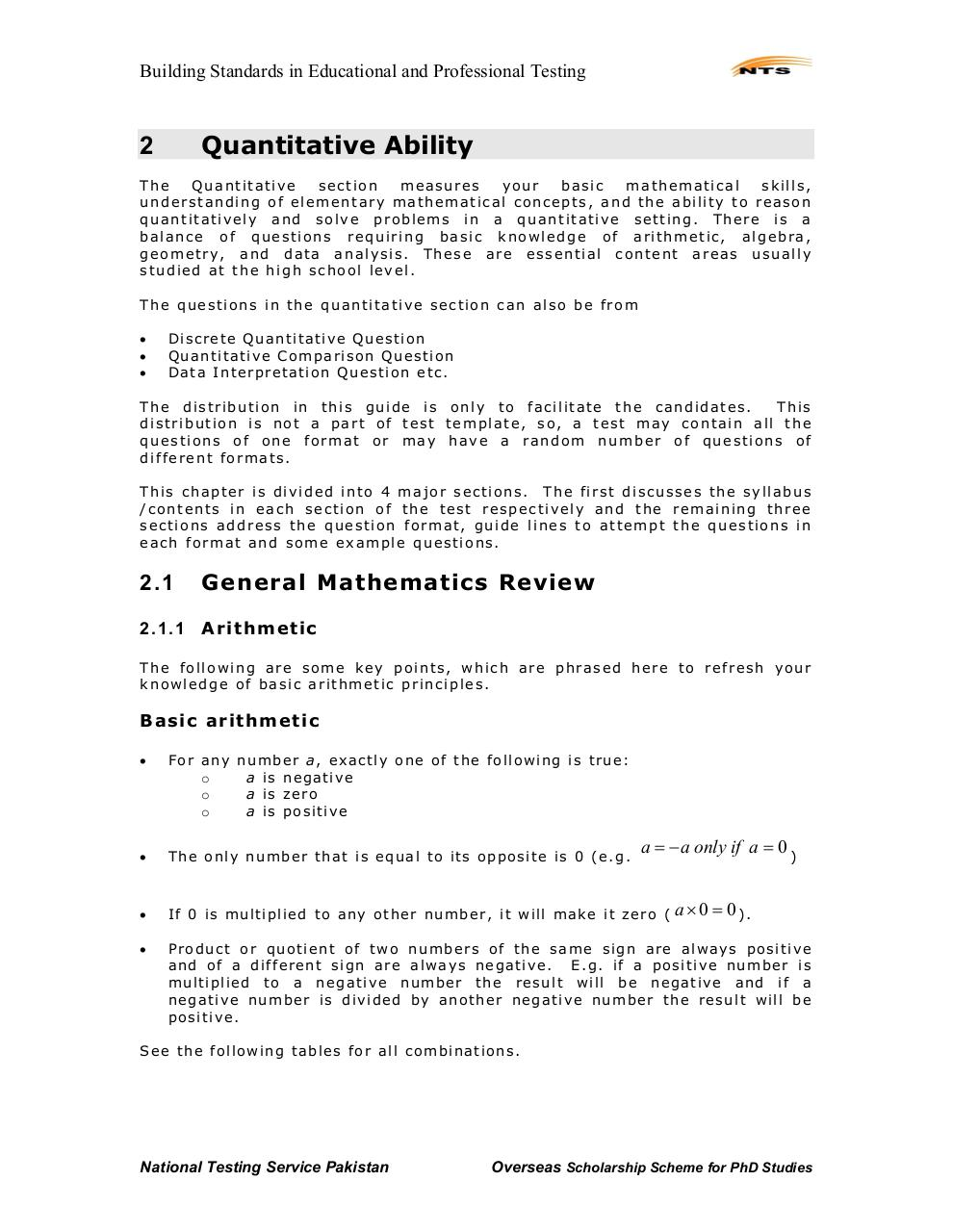# NTS Book for GAT General.pdfPage 1 2 345142

#### Text preview

Building Standards in Educational and Professional Testing

Quantitative Ability

2

The
Quantitative
section
measures
your
basic
mathematical
skills,
understanding of elementary mathematical concepts, and the ability to reason
quantitatively and solve problems in a quantitative setting. There is a
balance of questions requiring basic knowledge of arithmetic, algebra,
geometry, and data analysis. These are essential content areas usually
studied at the high school level.
The questions in the quantitative section can also be from

Di screte Quanti tative Questi on
Quanti tative Compari son Questi on
Data Interpretati on Questi on etc.

The distribution in this guide is only to facilitate the candidates.
This
distribution is not a part of test template, so, a test may contain all the
questions of one format or may have a random number of questions of
different formats.
This chapter is divided into 4 major sections. The first discusses the syllabus
/contents in each section of the test respectively and the remaining three
sections address the question format, guide lines to attempt the questions in
each format and some example questions.

2.1

General Mathematics Review

2.1.1

Arithmetic

The following are some key points, which are phrased here to refresh your
knowledge of basic arithmetic principles.

Basic arithmetic

For any number a, exactl y one of the fol l owi ng i s true:
o
a i s n egativ e
o
a i s z ero
o
a i s posi ti ve

Th e on l y nu m ber th at i s equ al to i ts opposi te i s 0 (e. g.

If 0 i s m ul ti pli ed to any oth er n um ber, i t will m ak e i t zero ( a × 0 = 0 ) .

a = − a only if a = 0 )

Product or quoti ent of two numbers of t h e same si gn are al ways posi ti ve
and of a d i fferent si gn are al ways negati ve. E. g. i f a po si ti ve number i s
mul ti pli ed to a negati ve number the r esul t wi ll be nega ti ve and i f a
negati ve number i s d i vi ded by another ne gati ve number the r esul t will be
posi ti ve.

S ee th e f oll owi n g tables f or all com bi n ati ons.

National Testing Service Pakistan

Overseas Scholarship Scheme for PhD Studies